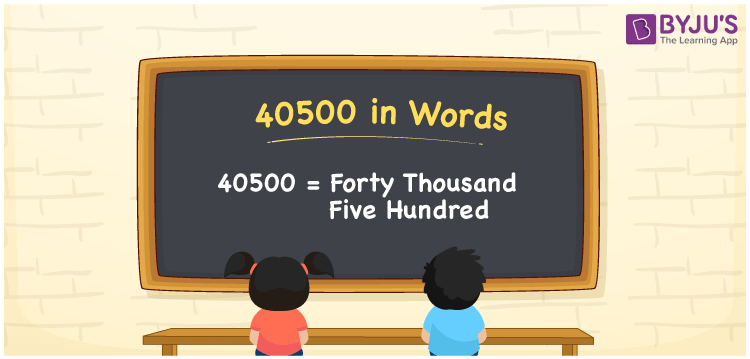# 40500 in Words

The number 40500 in words is “Forty Thousand Five Hundred”. Converting the numbers into words is very easy if you know the numeral system. It means that the place value system is very helpful to write 40500 in words. For example, if the price of the TV is Rs. 40500, it can be written as “The price of the TV is Rs. Forty Thousand Five Hundred. Now, let us have a look at the spelling of 40500 in English words with a complete explanation.

 40500 in Words: Forty Thousand Five Hundred. Forty Thousand Five Hundred in Numerical Form: 40500.

## 40500 in English Words

The number 40500 in English words is “Forty Thousand Five Hundred”.## How to Write 40500 in Words?

We can use the place value table to write 40500 in words. The following is the place value table for the number 40500.

 Ten – Thousands Thousands Hundreds Tens Ones 4 0 5 0 0

We can write the expanded form of 40500 as follows:

= 4 × Ten thousand + 0 × Thousand + 5 × Hundred + 0 × Ten + 0 × One

= 4 × 10000 + 5 × 100

= 40000 + 500

= 40500

= forty thousand five hundred

Hence, 40500 in words is forty thousand five hundred.

The number 40500 is a natural number that lies between 40499 and 40501.

40500 in words – Forty thousand five hundred

Is 40500 an odd number? – No

Is 40500 an even number? – Yes

Is 40500 a perfect square number? – No

Is 40500 a perfect cube number? – No

Is 40500 a prime number? – No

Is 40500 a composite number? – Yes

## Frequently Asked Questions on 40500 in Words

Q1

### How to spell 40500?

40500 in words is forty thousand five hundred.

Q2

### Simplify 40000 + 500, and express the value in words.

Simplifying 40000 + 500, we get 40500. Hence, 40500 in words is forty thousand five hundred.

Q3

### Is 40500 a prime number?

No, 40500 is not a prime number.